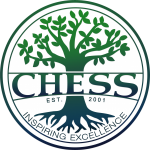## What students should be doing to prepare to take Algebra 1

Work on a solid foundation before starting algebra:

a. All students must have a working knowledge of adding, subtracting, multiplying, and dividing fractions.
b. Students must understand how to get percentages.
c. Students should be able to convert back and forth between fractions, decimals, and percentages.

These concepts must be learned before starting Algebra 1 and all high school science classes. Algebra 1 & 2, Geometry, and high school sciences all have fractions, percentages, and decimals and assume the student knows these concepts and does not teach them. In these high school textbooks, there is no side bar reminding you how to turn your fraction into a decimal or a percent. Students must remember.

Basic math is what often keeps the students from doing well in algebra, chemistry, and physics. So before starting algebra be sure the student is well practiced in fractions, decimals, and percentages.

## What students should be doing to prepare to take Pre-Algebra

Be sure students have covered percentages, fractions, decimals and converting between the three at least once. Pre-Algebra should not be the first time the student has learned this material. Pre-Algebra should be the last time this is formally taught.

There is hardly any difference between Math 6, Math 7, and Math 8. That does not mean the student should skip math 7 and math 8 because the student got it down in Math 6. Every time the material is repeated, the student learns the material better.

Students’ brains are growing as well as their body. They may have learned something last year, and act like they never saw it before the next year. Keep repeating the material until the student is ready for algebra.

CHESS meets at 8730 Sudley Road, Manassas, in the “Rock” building next to Manassas Baptist Church.About us

Contact

News & Events